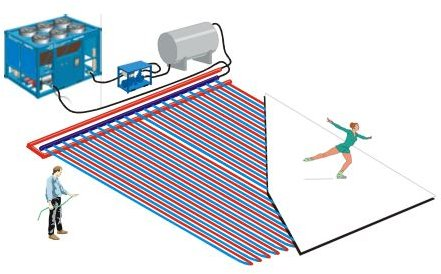# Refrigeration Loads Calculation for an Ice Rink – How to Calculate the Refrigeration Loads for an Ice Rink

The refrigeration load calculation is affected by the construction of the ice rink at large apart from the surrounding environment.

## Construction of a Typical Ice Rink• The bottom-most layer of the ice rink floor is made up of highly compacted sand with hot water pipes running across the layer. The purpose of this layer is to maintain a constant ground temperature in order to prevent any dislocation.
• One layer of insulating material is laid just above the sand layer so that the heat from the sand layer is not transferred above.
• A layer of concrete is laid just above the insulation layer; the cooling tube carrying refrigerant (evaporator) is embossed inside the concrete slab.
• The ice slab is deposited over this concrete slab.

## Some Theory of Refrigeration Load Calculation for Ice Rink

• First, we will determine the refrigeration load required to cool down the waters from the ambient temperature to zero degrees centigrade.
• Then, we will calculate the latent heat require to freeze the water to ice.
• After that, we will calculate the refrigeration load require to cool down the ice further to the desired temperature (say -4 degree centigrade).
• Next, we will calculate the refrigeration load reducing the cooling of the ice by means of conduction and convection by the ice and surrounding air.
• Finally, we will add up all the refrigeration loads to determine the total refrigeration load of the ice rink.

## Steps for Refrigeration Load Calculation for Ice Rink

• Let’s assume the following:

– Size of the ice rink = 10 m (l) X 5 m (b) X 0.5m (t)

– Density of ice (d)= 0.9 gm/cc = 900 kg/cubic metre

– Time duration for converting water to ice (T) = 5 hrs = 18000 sec.

– Ambient temperature (Tamb) = 20 degree C

– Final temperature of ice (Tice) = -4 degree C

– So, the weight of ice (M) = 22500 Kg.

– Specific heat of water (Swater) = 4186 J/kg -degree C

– Specific heat of ice (Sice) = 2093 J/kg -degree C

– Specific latent heat for fusion for ice (L) = 334000 J/Kg

– Thermal conductivity of ice (Kice) = 2.25 W/m-K

– Convection heat transfer coefficient for air (Uair) = 50 W/m2-K

• Refrigeration load required to cool down the water from ambient temperature to zero degree centigrade: (R1) = M * Swater * (Tamb – 0) = 1883700 J.
• Refrigeration load required to convert the zero degree water to zero degree ice: (R2) = M*L = 7515000 J.
• Refrigeration load required to cool down the ice further to – 4 degree centigrade: (R3) = M*Sice*(0-Tice) = 188370 J.
• Now the time required for the above three process is say 5 hrs. (18000 Sec.). So, the cumulative refrigeration load for the above three process KW will be R4 = (R1+R2+R3)/ (T*1000) = 532.615 KW.
• In order to calculate the refrigeration load (R5) required to prevent the ice from loosing its cool, we first need to calculate the overall heat transfer coefficient (U) of the ice and air:

U = 1 / [(1/Uair) + (t / Kice)] = 4.128440367 W / M2 – K

And,

R5 = [U * l * b * (Tamb – Tice)]/1000 = 4.954 KW

• So the final refrigeration load (R)for the ice rink is = R4 + R5 = 537.569 KW

## Conclusion

The example and the methodology discussed here for the refrigeration load calculation for ice rinks is good enough to start a practical calculation, but many other factors may come into the picture when dealing with different ice rinks’ refrigeration load calculations. Also, before actually doing any ice rink design, it is recommended to use the FEA tools available in the market to cross-check the results.

Image Courtesy

Wikipedia